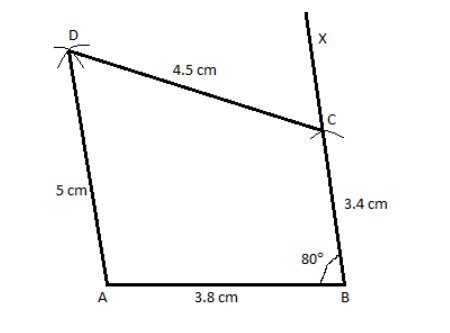Courses
Courses for Kids
Free study material
Free LIVE classes
MoreLIVE
Join Vedantu’s FREE Mastercalss

# Construct a quadrilateral ABCD in which the sides; AB = 3.8 cm, BC = 3.4 cm, CD = 4.5 cm, AD = 5 cm and the angle ∠B = 80°.Verified
335.1k+ views
Hint: In order to construct a quadrilateral with the given requirements of lengths of sides and an angle, we make a construction using the appropriate geometrical equipment. We make use of a ruler to measure the length of sides and a protractor to measure and draw an angle.

Given Data:
AB = 3.8 cm
BC = 3.4 cm
CD = 4.5 cm
∠B = 80°

The steps of construction to construct the quadrilateral ABCD are as follows:
First we draw a line segment of length 3.8 cm and name it ‘AB’.
Then with the point B as center we make a mark at 80° with respect to the horizontal AB and draw a line BX passing through the mark we made.
Now from point B we measure a length of 3.4 cm along the line segment BX and mark the point as C.
Now with A as the center and a radius of 5 cm we draw an arc.
Now with C as the center and radius equal to 4.5 cm, we draw another arc, cutting the previous arc and we name this point as D.
Now we join the line segments AD and CD respectively.
Thus the quadrilateral ABCD is obtained.
The figure obtained after we finish construction is as follows:Note: In order to solve this type of problems the key is to know how to construct a geometrical figure with a set of given requirements, using the help of geometrical apparatus. We measure a length of straight lines using a ruler, we measure angles using a protractor and we draw arcs or construct a circle using a compass. We find all these instruments in a geometrical box and each instrument has a specific use case. We do the construction in a stepwise manner.
Last updated date: 25th Sep 2023
Total views: 335.1k
Views today: 6.35k